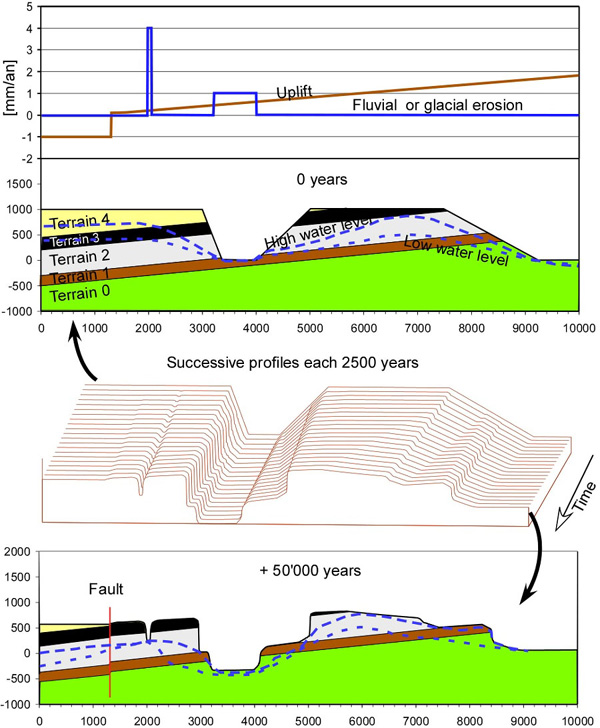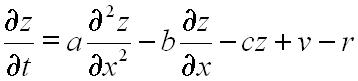Using the following equation a landscape submitted to uplifts is erodedwhere

z: altitude
x: abscise
t: temps
a: coefficient of diffusivity [mm/an],
b: coefficient of regressive erosion [mm/an]
c: coefficient de denudation [mm/an].
v: ” uplift ” velocity
r: velocity of fluvial or glacial erosion [mm/an].

The upper part of the figure represents the initial state, the lower the final, and in between the landscape evolution.